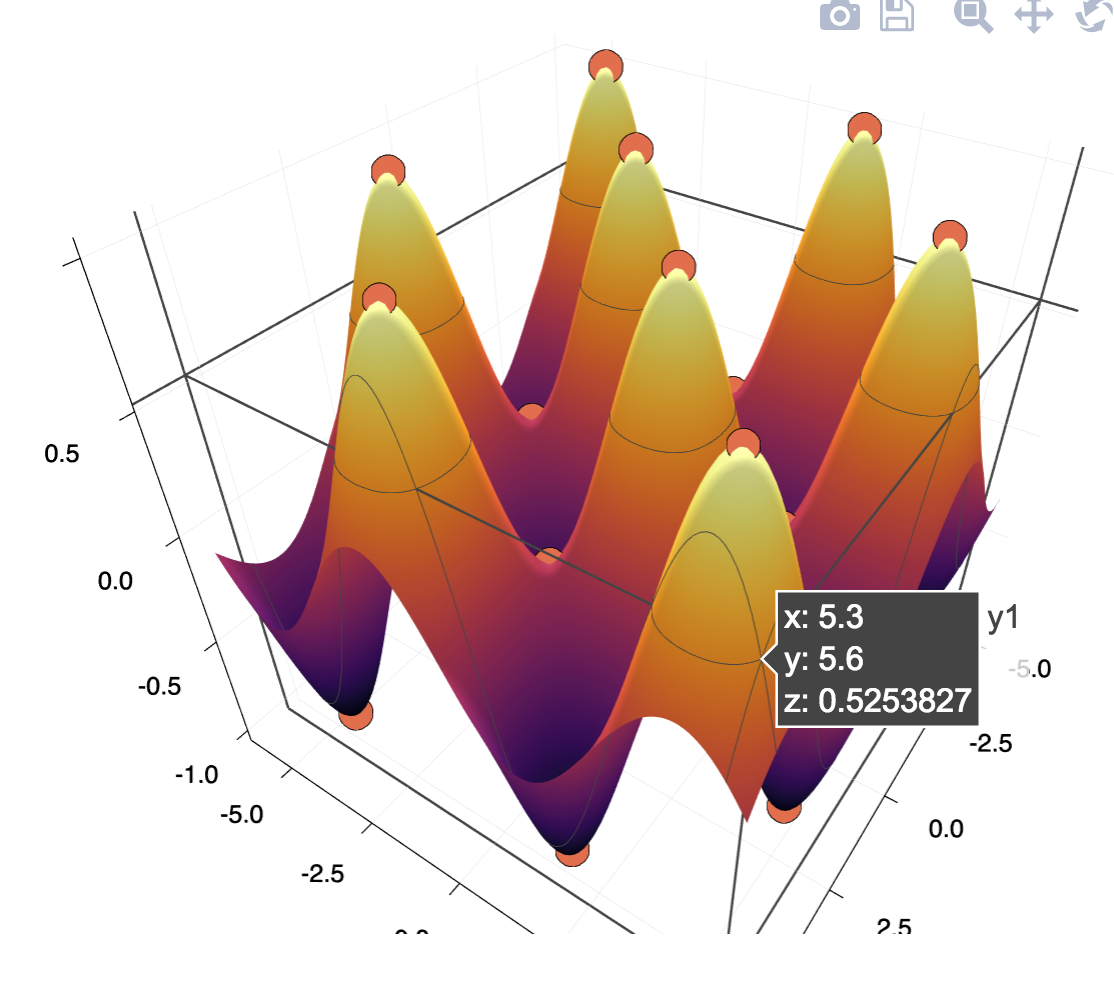# IntervalRootFinding.jl

This package provides guaranteed methods for finding roots of functions $f: \mathbb{R}^n \to \mathbb{R}^n$ with $n \ge 1$, i.e. vectors (or scalars, for $n=1$) $\mathbb{x}$ for which $f(\mathbb{x}) = \mathbb{0}$. In principle, it guarantees to find all roots inside a given box in $\mathbb{R}^n$, or report subboxes for which it is unable to provide guarantees.

To do so, it uses methods from interval analysis, using interval arithmetic from the IntervalArithmetic.jl package by the same authors.

Warning

While this package aimed at providing guaranteed results and despite our best efforts and test suite, some bugs may remain and there are several known issues with corner cases. Please look at the issue tracker and report there any odd and/or incorrect behavior.

## Basic 1D example

To begin, we need a standard Julia function and an interval in which to search roots of that function. Intervals use the Interval type provided by the IntervalArithmetic.jl package and are generally constructed using the .. syntax, a..b representing the closed interval $[a, b]$.

When provided with this information, the roots function will return a vector of all roots of the function in the given interval.

Example:

julia> using IntervalArithmetic, IntervalRootFinding

julia> rts = roots(x -> x^2 - 2x, 0..10)
2-element Array{Root{Interval{Float64}},1}:
Root([1.99999, 2.00001], :unique)
Root([0, 4.4724e-16], :unknown)

The roots are returned as Root objects, containing an interval and the status of that interval, represented as a Symbol. There are two possible types of root status, as shown in the example:

• :unique: the given interval contains exactly one root of the function,
• :unknown: the given interval may or may not contain one or more roots; the algorithm used was unable to come to a conclusion.

The second status is still informative, since all regions of the original search interval not contained in any of the returned root intervals is guaranteed not to contain any root of the function. In the above example, we know that the function has no root in the interval $[2.1, 10]$, for example.

There are several known situations where the uniqueness (and existence) of a solution cannot be determined by the interval algorithms used in the package:

• If the solution is on the boundary of the interval (as in the previous example);
• If the derivative of the solution is zero at the solution.

In particular, the second condition means that multiple roots cannot be proven to be unique. For example:

julia> g(x) = (x^2 - 2)^2 * (x^2 - 3)
g (generic function with 1 method)

julia> roots(g, -10..10)
4-element Array{IntervalRootFinding.Root{IntervalArithmetic.Interval{Float64}},1}:
Root([1.73205, 1.73206], :unique)
Root([1.41418, 1.4148], :unknown)
Root([-1.4148, -1.41418], :unknown)
Root([-1.73206, -1.73205], :unique)

Here we see that the two double roots are reported as being possible roots without guarantee and the simple roots have been proved to be unique.

## Basic multi-dimensional example

For dimensions $n > 1$, the function passed to roots must currently return an SVector from the StaticArrays.jl package.

Here we give a 3D example:

julia> function g( (x1, x2, x3) )
return SVector(x1^2 + x2^2 + x3^2 - 1,
x1^2 + x3^2 - 0.25,
x1^2 + x2^2 - 4x3
)
end
g (generic function with 1 method)

julia> X = -5..5
[-5, 5]

julia> rts = roots(g, X × X × X)
4-element Array{Root{IntervalBox{3,Float64}},1}:
Root([0.440762, 0.440763] × [0.866025, 0.866026] × [0.236067, 0.236068], :unique)
Root([0.440762, 0.440763] × [-0.866026, -0.866025] × [0.236067, 0.236068], :unique)
Root([-0.440763, -0.440762] × [0.866025, 0.866026] × [0.236067, 0.236068], :unique)
Root([-0.440763, -0.440762] × [-0.866026, -0.866025] × [0.236067, 0.236068], :unique)

Thus the system admits four unique roots in the box $[-5, 5]^3$. We have used the unicode character × (typed as \times<tab>) to compose several intervals into a multidimensional box.

## Stationary points

Stationary points of a function $f:\mathbb{R}^n \to \mathbb{R}$ may be found as zeros of the gradient of $f$. The package exports the ∇ operator to calculate gradients using ForwardDiff.jl:

julia> f( (x, y) ) = sin(x) * sin(y)
f (generic function with 1 method)

julia> ∇f = ∇(f)  # gradient operator from the package
(::#53) (generic function with 1 method)

julia> rts = roots(∇f, IntervalBox(-5..6, 2), Newton, 1e-5)
25-element Array{IntervalRootFinding.Root{IntervalArithmetic.IntervalBox{2,Float64}},1}:
Root([4.71238, 4.71239] × [4.71238, 4.71239], :unique)
Root([4.71238, 4.71239] × [1.57079, 1.5708], :unique)
⋮
[output snipped for brevity]

Now let's find the midpoints and plot them:

midpoints = mid.(interval.(rts))

xs = first.(midpoints)
ys = last.(midpoints)

using Plots; plotlyjs()

surface(-5:0.1:6, -6:0.1:6, (x,y) -> f([x, y]))
scatter!(xs, ys, f.(midpoints))

The result is the following: